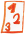Math worksheetsCustom math worksheets at your fingertips

# Details for problem "Expand and simplify binomic formula"

Quickname: 7813

## Summary

Apply binomic formula expansion rules to simplify term.

## Example## Description

Apply the well known binomic formula expansion rules to expand and simplify terms. The summands of the formula's factors will be either a variable and a literal number or both of them will be variables. In no case, both summands will be numbers, which means the expanded term will always contain variables and it does not simplify to a single literal number. The number of problems is selectable. For the types of formulas that will appear it can be specified that only some of the three binomic formulas will be used. Variables that appear will always be named a and b or x and y.

Download free worksheets for this math problem here. There is a second sheet with the solutions. Just click on the respective link.Worksheet 1Solution sheetWorksheet 2Solution sheetWorksheet 3Solution sheet

If you can not see the solution sheets for download, they may be filtered out by an ad blocker that you may have installed. If this is the case, please allow ads for this page and reload the page. The solution sheets will then reappear.

• Do the sample worksheets do not really fit?
• Do you need more math worksheets, with a different level of difficulty?
• Would you like to combine different problems on a worksheet and adjust them to your needs?
• As a teacher, you can put together your own worksheets using the automatically generated math problems provided.
With a free initial credit, you can start creating your own math worksheets in a few minutes.

It does not cost anything to try! Register here, to create custom worksheets now!

## Customization options for this problem

ParameterPossible values
Number of problems1, 2, 3, 4, 5, 6, 7, 8, 9, 10
Binomic formula types(a+b)^2, (a-b)^2, (a+b)(a-b), (a+b)^2 & (a-b)^2, all

## Similar problems

fill in blank spaces in binomic formulasFill gaps correctly in binomic formulasBinomic formula fill in blanks
get to know the elements of binomic formulasElements of a binomic formula and their expanded form are grouped in a table. Fill the empty spaces.Examine the elements of binomic formulas
simpler versionApply binomic formula expansion rules to simplify term. Advanced version mit multiples of variables as summands.Expand and simplify binomic formula, advanced
matching problem: Find correct pairs of binomic formulas and expanded termsFind matching terms of original and simplified binomic formulas in two lists.Match binomic formula to simplified form
expand and simplify a simple termExpand product term with variables.Product term expansion
where binomic formula is embedded in other termSimplify a term which contains a binomic formulaSimplify term with binomic formula, combine like terms
the other way round: Specify the binomic formulaA term is given which is the expanded form of a binomic formula. The original binomic formula has to be found.Specify binomic formula for expanded term

Deutsche Version dieser Aufgabe
These informational pages with samples describe math problems that can be combined on custom math worksheets with solutions for home and school use.